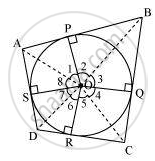# Prove that Opposite Sides of a Quadrilateral Circumscribing a Circle Subtend Supplementary Angles at the Centre of the Circle. - Mathematics

Sum

Prove that opposite sides of a quadrilateral circumscribing a circle subtend supplementary angles at the centre of the circle.

#### SolutionLet ABCD be a quadrilateral circumscribing a circle centered at O such that it touches the circle at point P, Q, R, S. Let us
join the vertices of the quadrilateral ABCD to the center of the circle.
Consider ΔOAP and ΔOAS,
AP = AS (Tangents from the same point)

OP = OS (Radii of the same circle)

OA = OA (Common side)

ΔOAP ≅ ΔOAS (SSS congruence criterion)

Therefore, A ↔ A, P ↔ S, O ↔ O

And thus, ∠POA = ∠AOS

∠1 = ∠8
Similarly,

∠2 = ∠3

∠4 = ∠5

∠6 = ∠7

∠1 + ∠2 + ∠3 + ∠4 + ∠5 + ∠6 + ∠7 + ∠8 = 360º

(∠1 + ∠8) + (∠2 + ∠3) + (∠4 + ∠5) + (∠6 + ∠7) = 360º

2∠1 + 2∠2 + 2∠5 + 2∠6 = 360º

2(∠1 + ∠2) + 2(∠5 + ∠6) = 360º

(∠1 + ∠2) + (∠5 + ∠6) = 180º

∠AOB + ∠COD = 180º

Similarly, we can prove that ∠BOC + ∠DOA = 180º
Hence, opposite sides of a quadrilateral circumscribing a circle subtend supplementary angles at the centre of the circle.

Concept: Number of Tangents from a Point on a Circle
Is there an error in this question or solution?